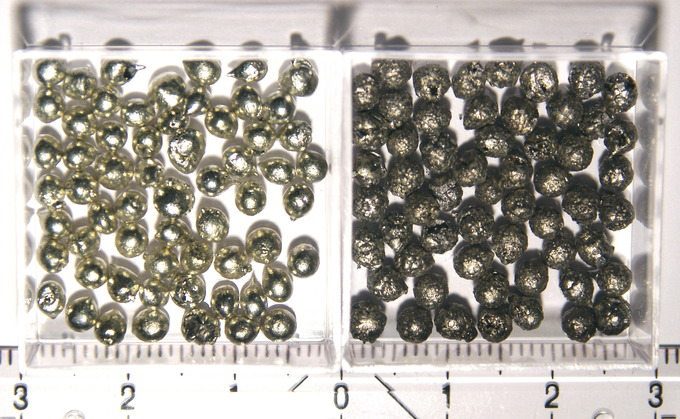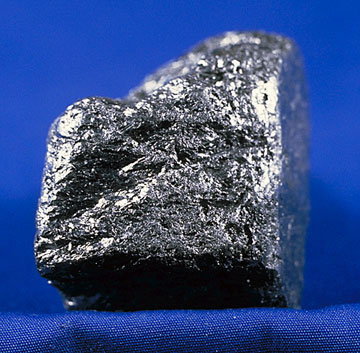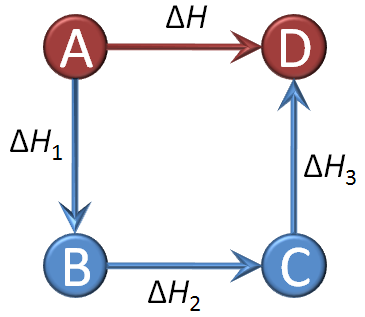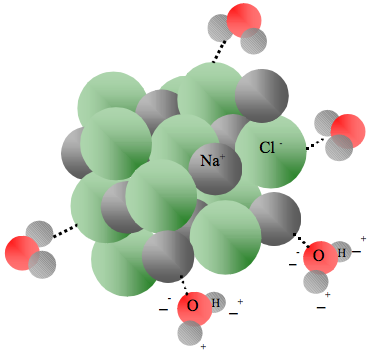## Standard States and Standard Enthalpy Changes

The standard enthalpy of formation refers to the enthalpy change when one mole of a compound is formed from its elements.

### Learning Objectives

Calculate the standard enthalpy of formation

### Key Takeaways

#### Key Points

• The standard state of a material is a reference point for the material’s thermodynamic state properties such as enthalpy, entropy, Gibbs free energy, etc. It is used to calculate the material’s properties under different conditions and is denoted as $H^\ominus_f$.
• The standard state for a gas is the hypothetical state at 1 bar; for liquids and solids, the pure substance at 1 bar; for elements, the most stable allotrope of the element; and for a substance in solution (solute), concentration at 1 M and 1 bar.
• The standard enthalpy of formation is the change in enthalpy that accompanies the formation of one mole of the compound from its elements. The standard enthalpy of reaction occurs in a system when one mole of matter is transformed by a chemical reaction.

#### Key Terms

• standard state: In chemistry, a reference point used to calculate a material’s (pure substance, mixture, or solution) properties under different conditions.
• standard enthalpy of formation: The change in enthalpy that accompanies the formation of one mole of a compound from its elements, with all substances in their standard states; also called “standard heat of formation.”
• enthalpy of solution: The heat association with dissolving a particular solute in a particular solvent.

### Standard States

In chemistry, the standard state of a material, be it a pure substance, mixture, or solution, is a reference point used to calculate its properties under different conditions. In principle, the choice of standard state is arbitrary, although the International Union of Pure and Applied Chemistry (IUPAC) recommends a conventional set of standard states for general use. A standard pressure of 1 bar (101.3 kilopascals) has been accepted.

Strictly speaking, temperature is not part of the definition of a standard state; the standard state of a gas is conventionally chosen to be 1 bar for an ideal gas, regardless of the temperature. However, most tables of thermodynamic quantities are compiled at specific temperatures, most commonly 298.15 K (exactly 25°C) or, somewhat less commonly, 273.15 K (exactly 0°C).

Standard states for atomic elements are given in terms of the most stable allotrope for each element. For example, white tin and graphite are the most stable allotropes of tin and carbon, respectively. Therefore, they are used as standard states or reference points for calculations of different thermodynamic properties of these elements.Tin: White tin (on the left) is the most stable allotrope of tin, and is used as its standard state for thermodynamic calculations.

The standard state should not be confused with standard temperature and pressure (STP) for gases, or with the standard solutions used in analytical chemistry. Standard states are often indicated in textbooks by a circle with a horizontal bar $H^\ominus_f$.Graphite: Graphite is the most stable state of carbon and is used in thermochemistry to define the heat of formation of carbon compounds.

### Standard Enthalpy of Formation

The standard enthalpy of formation, or standard heat of formation, of a compound is the change in enthalpy that accompanies the formation of one mole of the compound from its elements in their standard states. For example, the standard enthalpy of formation for carbon dioxide would be the change in enthalpy for the following reaction:

$C(s)(\text{graphite})+\text{O}_2(g)\rightarrow\text{CO}_2(g)\quad\quad\quad\quad \Delta H^\ominus_f=-394\text{ kJ/mol}$

Note that standard enthalpies of formation are always given in units of kJ/mol of the compound formed.

## Standard Enthalpy of Reaction

The standard enthalpy of reaction is the enthalpy change that occurs in a system when a chemical reaction transforms one mole of matter under standard conditions.

### Learning Objectives

Demonstrate how to directly calculate the standard enthalpy of reaction

### Key Takeaways

#### Key Points

• The standard enthalpy of reaction, $\Delta H^\ominus _{rxn}$, can be calculated by summing the standard enthalpies of formation of the reactants and subtracting the value from the sum of the standard enthalpies of formation of the products.
• The following equation can be used to calculate the standard enthalpy of reaction: $\Delta H^\ominus _{rxn}=\sum \Delta H^\ominus _f\{\text{products}\}-\sum \Delta H^\ominus _f\{\text{reactants}\}$.
• The enthalpy of reaction is calculated under standard conditions (STP).

#### Key Terms

• standard enthalpy of reaction: The enthalpy change that occurs in a system when one mole of matter is transformed by a chemical reaction under standard conditions.

The standard enthalpy of reaction, $\Delta H^\ominus _{rxn}$, is the change in enthalpy for a given reaction calculated from the standard enthalpies of formation for all reactants and products. The change in enthalpy does not depend upon the particular pathway of a reaction, but only upon the overall energy level of the products and reactants; enthalpy is a state function, and as such, it is additive. In order to calculate the standard enthalpy of a reaction, we can sum up the standard enthalpies of formation of the reactants and subtract this from the sum of the standard enthalpies of formation of the products. Stated mathematically, this gives us:

$\Delta H^\ominus _{rxn}=\sum \Delta H^\ominus _f\{\text{products}\}-\sum \Delta H^\ominus _f\{\text{reactants}\}$

### Calculating the Standard Enthalpy of Reaction

Calculate the standard enthalpy of reaction for the combustion of methane:

$\text{CH}_4(g)+2\text{O}_2(g)\rightarrow\text{CO}_2(g)+2\text{H}_2\text{O}(g)\quad\quad \Delta H^\ominus _{rxn}=?$

In order to calculate the standard enthalpy of reaction, we need to look up the standard enthalpies of formation for each of the reactants and products involved in the reaction. These are typically found in an appendix or in various tables online. For this reaction, the data we need is:

$\Delta H^\ominus _f\{\text{CH}_4(g)\}=-75\text{ kJ/mol}$

$\Delta H^\ominus _f\{\text{O}_2(g)\}=0\text{ kJ/mol}$

$\Delta H^\ominus _f\{\text{CO}_2(g)\}=-394\text{ kJ/mol}$

$\Delta H^\ominus _f\{\text{H}_2\text{O}(g)\}=-284\text{ kJ/mol}$

Note that because it exists in its standard state, the standard enthalpy of formation for oxygen gas is 0 kJ/mol. Next, we sum up our standard enthalpies of formation. Keep in mind that because the units are in kJ/mol, we need to multiply by the stoichiometric coefficients in the balanced reaction equation.

$\begin{array}{rcl}\sum\Delta H^\ominus _f\{\text{products}\}\,\,&=&\Delta H^\ominus _f\{\text{CO}_2(g)\}+\Delta H^\ominus _f\{\text{H}_2\text{O}(g)\}\\{}&=&(1)(-394)+(2)(-284)=-962\text{ kJ/mol}\end{array}$

$\begin{array}{rcl}\sum\Delta H^\ominus _f\{\text{reactants}\}&=&\Delta H^\ominus _f\{\text{CH}_4(g)\}+\Delta H^\ominus _f\{\text{O}_2(g)\}\\{}&=&(1)(-75)+(2)(0)=-75\text{ kJ/mol}\end{array}$

Now, we can find the standard enthalpy of reaction:

$\begin{array}{rcl}\Delta H^\ominus _{rxn}&=&\sum \Delta H^\ominus _f\{\text{products}\}-\sum \Delta H^\ominus _f\{\text{reactants}\}\\{}&=&(-962)-(-75)=-887\text{ kJ/mol}\end{array}$

As we would expect, the standard enthalpy for this combustion reaction is strongly exothermic.

A calculation of standard enthalpy of reaction (∆H°rxn) from standard heats of formation (∆H°f): A standard enthalpy of reaction (∆H°rxn) problem, involving ethylene and oxygen as reactants to yield carbon dioxide and gaseous water, is shown.

## Hess’s Law

Hess’s Law sums the changes in enthalpy for a series of intermediate reaction steps to find the overall change in enthalpy for a reaction.

### Learning Objectives

Employ Hess’s law to determine ΔH∘rxn

### Key Takeaways

#### Key Points

• Hess’s law states that the standard reaction enthalpy is the sum of the standard enthalpies of the intermediate reactions into which the overall reaction can be divided, while each occurs at the same temperature.
• Enthalpy change for a reaction is independent of the number of ways a product can be obtained, if the initial and final conditions are the same.
• Negative enthalpy change for a reaction indicates exothermic process, while positive enthalpy change corresponds to endothermic process.

#### Key Terms

• Hess’s law: States that, if an overall reaction takes place in several steps, its standard reaction enthalpy is the sum of the standard enthalpies of the intermediate reactions, at the same temperature.
• law of conservation of energy: States that the total amount of energy in any isolated system remains constant; energy cannot be created or destroyed, although it can change forms.

### Derivation of Hess’s Law

Hess’s law is a relationship in physical chemistry named after Germain Hess, a Swiss-born Russian chemist and physician. This law states that if a reaction takes place in several steps, then the standard reaction enthalpy for the overall reaction is equal to the sum of the standard enthalpies of the intermediate reaction steps, assuming each step takes place at the same temperature.

Hess’s law derives directly from the law of conservation of energy, as well as its expression in the first law of thermodynamics. Since enthalpy is a state function, the change in enthalpy between products and reactants in a chemical system is independent of the pathway taken from the initial to the final state of the system. Hess’s law can be used to determine the overall energy required for a chemical reaction, especially when the reaction can be divided into several intermediate steps that are individually easier to characterize. Negative enthalpy change for a reaction indicates exothermic process, while positive enthalpy change corresponds to endothermic process.Graphical representation of Hess’s law: The net reaction here is A being converted into D, and the change in enthalpy for that reaction is ΔH. However, we can see that the net reaction is a result of A being converted into B, which is then converted into C, which is finally converted into D. By Hess’s law, the net change in enthalpy of the overall reaction is equal to the sum of the changes in enthalpy for each intermediate transformation: ΔH = ΔH1+ΔH2+ΔH3.

### Calculating Standard Enthalpies of Reaction Using Hess’s Law

$C(s)\{\text{graphite}\}\rightarrow C(s)\{\text{diamond}\}\quad\quad \Delta H_{rxn}=?$

Turning graphite into diamond requires extremely high temperatures and pressures, and therefore is impractical in a laboratory setting. The change in enthalpy for this reaction cannot be determined experimentally. However, because we know the standard enthalpy change for the oxidation for these two substances, it is possible to calculate the enthalpy change for this reaction using Hess’s law. Our intermediate steps are as follows:

$C(s)\{\text{graphite}\}+O_2(g)\rightarrow CO_2(g)\quad\quad \Delta H^\circ=-393.41\text{ kJ/mol}$

$C(s)\{\text{diamond}\}+O_2(g)\rightarrow CO_2(g)\quad\quad \Delta H^\circ=-395.40\text{ kJ/mol}$

In order to get these intermediate reactions to add to our net overall reaction, we need to reverse the second step. Keep in mind that when reversing reactions using Hess’s law, the sign of ΔH will change. Sometimes, you will need to multiply a given reaction intermediate through by an integer. In such cases, you need always multiply your ΔH value by that same integer. Restating the first equation and flipping the second equation, we have:

$C(s)\{\text{graphite}\}+O_2(g)\rightarrow CO_2(g)\quad\quad \Delta H^\circ=-393.41\text{ kJ/mol}$

$CO_2(g)\rightarrow C(s)\{\text{diamond}\}+O_2(g)\quad\quad\Delta H^\circ=+395.40\text{ kJ/mol}$

Adding these equations together, carbon dioxides and oxygens cancel, leaving us only with our net equation. By Hess’s law, we can sum the ΔH values for these intermediate reactions to get our final value, $\Delta H^\circ_{rxn}$.

$C(s)\{\text{graphite}\}\rightarrow C(s)\{\text{diamond}\}\quad\quad \Delta H^\circ_{rxn}=1.89\text{ kJ/mol}$

Hess’s law lesson: This lesson uses two methods to find the heat of reaction for a given reaction. First it looks at combining reactions according to Hess’s law and their heats of reaction, and then it discusses using standard heats of formation of the reactants and products to find the overall heat of reaction.

## Heat of Solution

Heat of solution refers to the change in enthalpy when a solute is dissolved into a solvent.

### Learning Objectives

Define heat of solution

### Key Takeaways

#### Key Points

• Enthalpy of solution, or heat of solution, is expressed in kJ/mol, and it is the amount of heat energy that is released or absorbed when a solution is formed.
• There are three steps in solvation: the breaking of bonds between solute molecules, the breaking of intermolecular attractions between solvent molecules, and the formation of new solute-solvent attractive bonds. Energy is absorbed during the first two steps, and it is released during the last step.
• Depending on the relative amounts of energy required to break bonds initially, as well as how much is released upon solute-solvent bond formation, the overall heat of solution can either be endothermic or exothermic.

#### Key Terms

• heat of solution: The enthalpy change associated with the dissolution of a substance in a solvent at constant pressure, resulting in infinite dilution.
• solvation: The process of attraction and association of molecules of a solvent with molecules or ions of a solute; also called dissolution.

The heat of solution, also referred to the enthalpy of solution or enthalpy of dissolution, is the enthalpy change associated with the dissolution of a solute in a solvent at constant pressure, resulting in infinite dilution. The heat of solution, like all enthalpy changes, is expressed in kJ/mol for a reaction taking place at standard conditions (298.15 K and 1 bar).

### Three-Step Process of Dissolution

The heat of solution can be regarded as the sum of the enthalpy changes of three intermediate steps:

1. The breaking of bonds within the solute, such as the electrostatic attraction between two ions (endothermic)
2. The breaking of intermolecular attractive forces within the solvent, such as hydrogen bonds (endothermic)
3. The formation of new attractive solute-solvent bonds in solution (exothermic)

The value of the overall heat of solution, $\Delta H^\circ_{sol}$, is the sum of these individual steps. Depending on the relative signs and magnitudes of each step, the overall heat of solution can be either positive or negative, and therefore either endothermic or exothermic. This depends entirely on if more energy was used to break the solute-solute and solvent-solvent bonds, or if more energy was released when solute-solvent bonds were formed.

If more energy is released in making bonds than is used in breaking bonds, the overall process is exothermic, and ∆Hsol is negative. If more energy is used in breaking bonds than is released upon solute-solvent bond formation, then the overall process is endothermic, and ∆Hsol is positive.

### Examples

• Dissolution of sodium chloride (table salt) in water is endothermic. This is because the amount of energy used to break apart the hydrogen bonding interactions between water molecules, as well as the energy used to break apart the electrostatic attractions between sodium and chloride ions, is greater than the amount of energy released when new solute-solvent attractions are formed between water molecules and aqueous ions in solution.
• Dissolving potassium hydroxide is exothermic. This is because more energy is released upon formation of solute-solvent bonds than was required to break apart the hydrogen bonds in water, as well as the ionic bonds in KOH.Dissolution of NaCl in water: Dissolution of sodium chloride in water is endothermic. Solute-solvent attractive bond formation (the exothermic step in the process of solvation) is indicated by dashed lines.